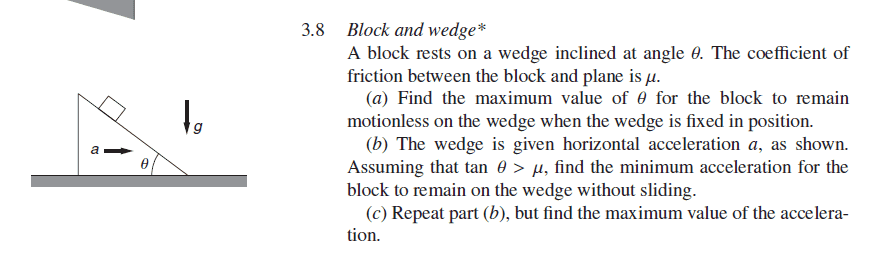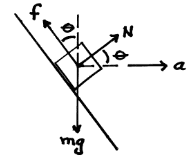# A block on an accelerating wedge

Gold Member
Homework Statement:
This is a statement.
Relevant Equations:
This is an equation.For question b, the official solution sets up a non-inertial coordinate on the block and writes out the following two equations:
\begin{cases} \begin{align*} f\cos(\theta)+N\sin(\theta)-mg=0 \qquad \hat\jmath \\ N\cos(\theta)+f\sin(\theta)=ma \qquad\quad\;\;\, \hat\imath \end{align*} \end{cases}The questions above are true because the horizontal acceleration of the block must be the same as the acceleration of the wedge to keep the former on the latter.

However, if I try to solve for the normal force using (2), I will obtain ##N=\frac{mg}{\mu \sin(\theta) + \cos(\theta)}## which shows that the magnitude of the normal force is independent to the acceleration.

This counterintuitive conclusion confuses me-- if you push it with a greater horizontal force, the block will be pinned harder to the ground because the block needs a stronger force to accelerate in lockstep with the wedge, and therefore creates a larger normal force. Could someone explain me why this is the case? Thanks in advance :).

Last edited:

## Answers and Replies

TSny
Homework Helper
Gold Member
When you solved for the normal force, you assumed that ##f = \mu N##. This is the value of ##f## when the acceleration has the specific value that puts the block on the verge of slipping. For general values of acceleration, you cannot assume ##f = \mu N##.

•Leo Liu
Gold Member
When you solved for the normal force, you assumed that ##f = \mu N##. This is the value of ##f## when the acceleration has the specific value that puts the block on the verge of slipping. For general values of acceleration, you cannot assume ##f = \mu N##.
So can you please tell me what the friction force will be if the block is not on the verge of slipping?

TSny
Homework Helper
Gold Member
So can you please tell me what the friction force will be if the block is not on the verge of slipping?
##f## and ##N## will depend on the acceleration of the system. When the block is not slipping, then you can use your two equations to solve for ##f## and ##N## for a given acceleration.

Gold Member
##f## and ##N## will depend on the acceleration of the system. When the block is not slipping, then you can use your two equations to solve for ##f## and ##N## for a given acceleration.
Oh I think I get it-- in this case the value of the acceleration will be constant, which means that we still have two variables to solve. Thank you!